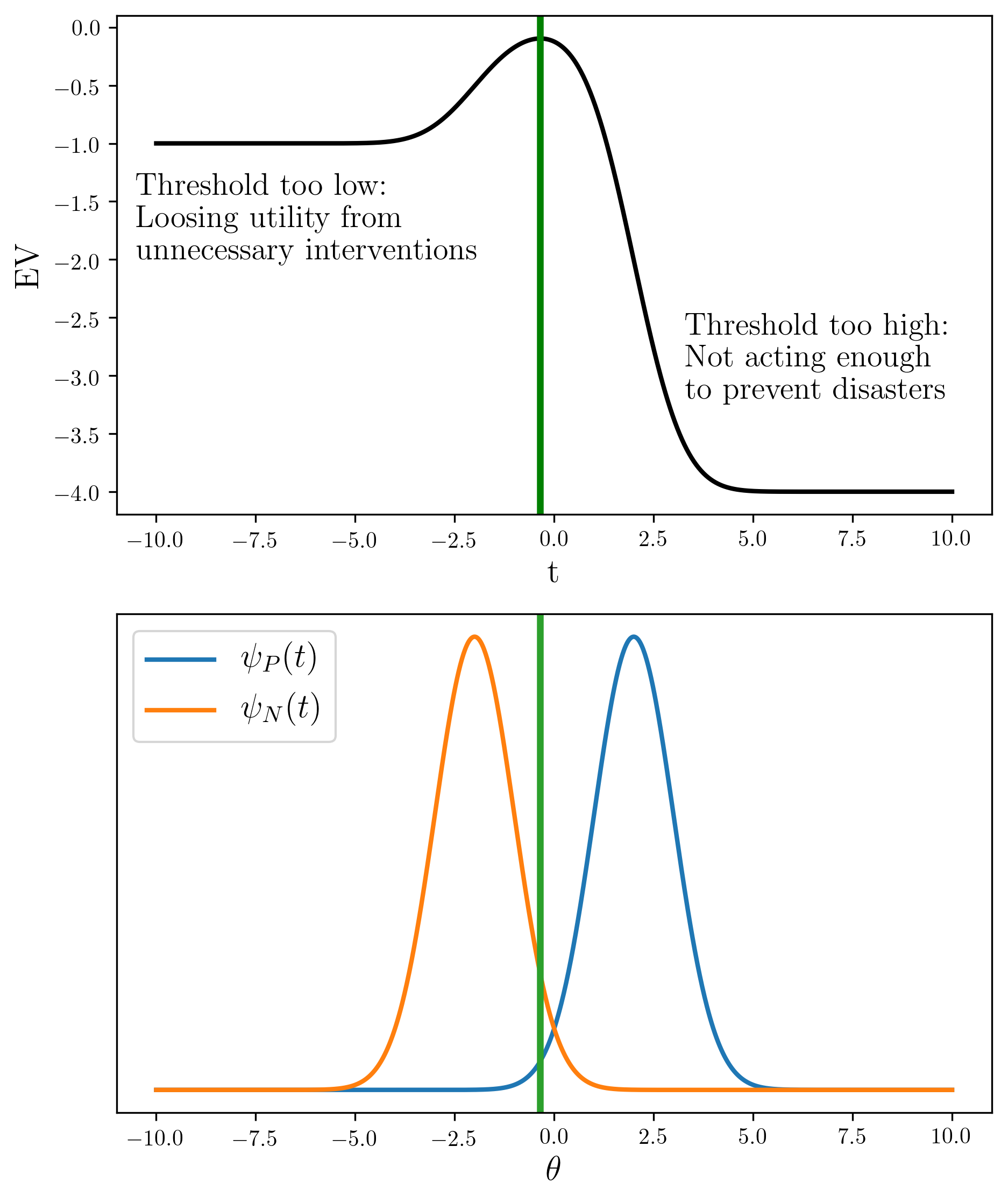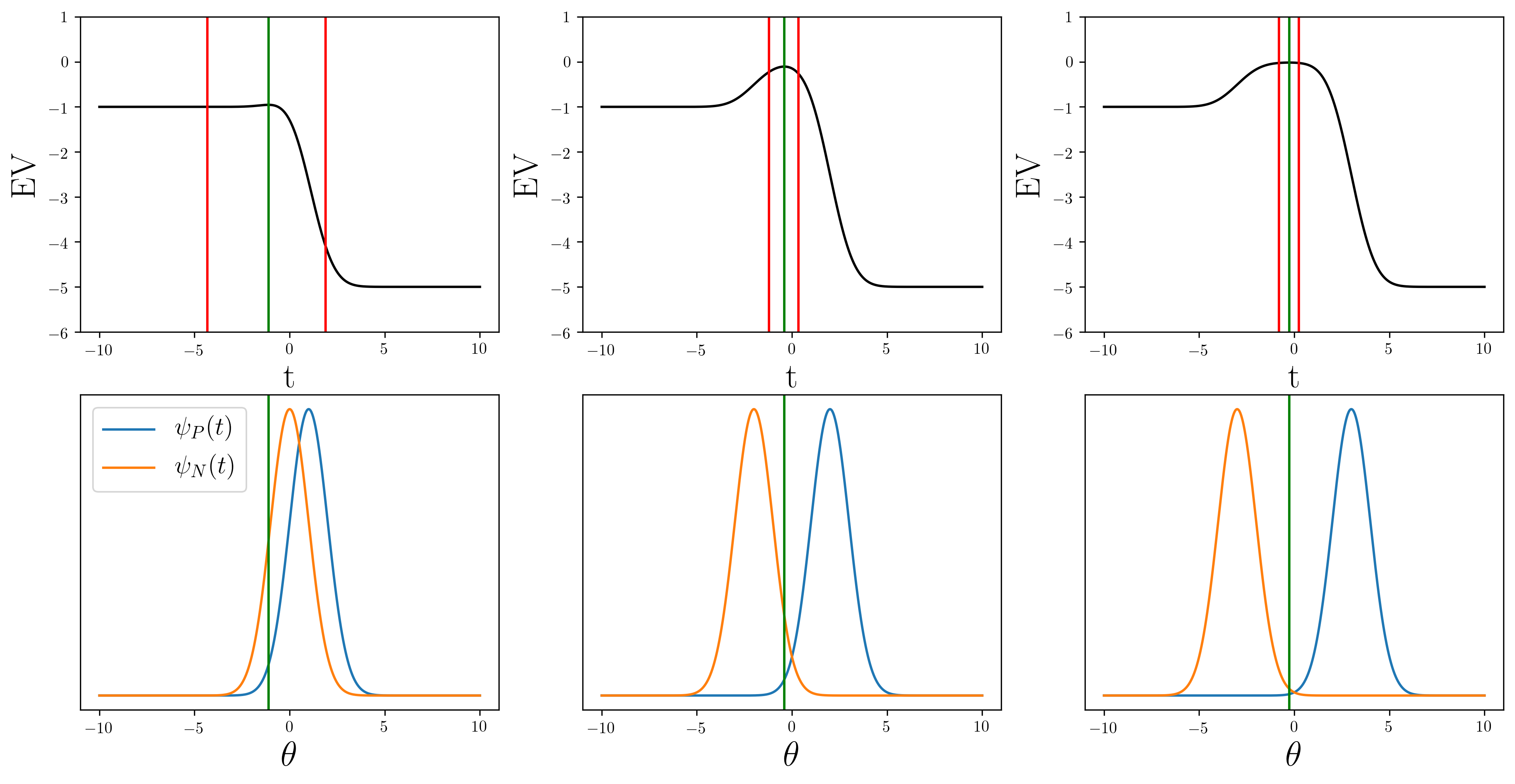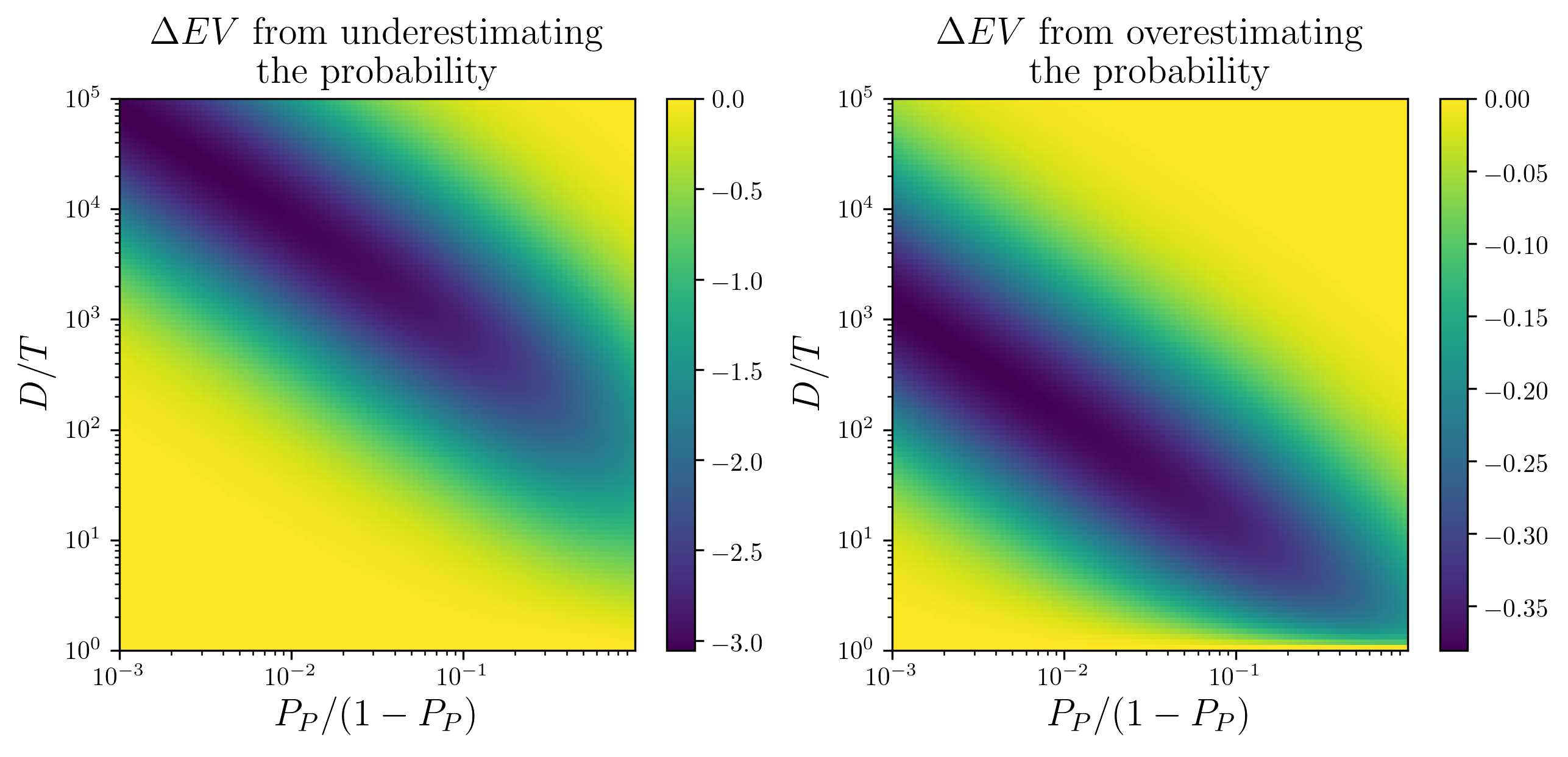# 40

In this post I consider a model where there is a possible bad outcome, and the chance of it happening is related to something we can measure. We need to be able to set a threshold for these measurements, such that if a measurement exceeds a threshold then we decide to act; to prepare for or treat the bad outcome. This seems like a pretty basic idea, so I expect that either most results will be trivial, or that someone has already done this before.

A basic example of this would be a disease, where if a patient reaches a threshold for the number and severity of symptoms then the doctor decides to prescribe a medicine. Or the current pandemic, where in February 2020 if governments had a more accurate view of both the chance and severity of a global pandemic they may have decided to put in place seemingly drastic measures to stop worse outcomes in the future.
This should also apply to important risks in the future, where it is important to know the probabilities and magnitudes so that we can adequately prepare. We need to know where to sensibly set the threshold which tells us when to act.

A helpful metaphor I will use here is a building with a sprinkler system. We want the sprinklers to turn on when there is a fire (there is a huge cost if the building burns down), but also we don't want the sprinklers constantly going off whenever there's dust in the air because this also has a cost associated with it.

When deciding whether something is going to happen, and whether to act there are 4 options:

• True Positive (TP), where the event happens but luckily we decided to act
• The building is on fire, but the sprinklers turned on and saved everything
• True Negative (TN), where nothing happens and we didn't do anything
• No fire, no sprinklers
• False Positive (FP), where nothing happens and we act unnecessarily
• The building is not on fire, but the sprinklers turned on anyway
• False Negative (FN), where the event happens and we didn't act
• The building is on fire, but the sprinklers didn't turn on and the building burned down

The chance of each of these options will depend on where we set our threshold. If the threshold is very low then we will have a lot of True Positives, but will also have to incur the costs of more False Positives. If the threshold is very high then we will have less False Positives, but will have more False Negatives.

## Mathematical Model

We can decide where to put the threshold by calculating the expected value () at a given threshold.

Where  is the probability of each outcome, and  is the return or value of each outcome.

We can express the value of each outcome quite simply, using 2 positive constants:
is the cost we have to pay if we don't treat the disease or avert the disaster
- The cost of the building burning down
is the cost of the treatment or the preparations
- The cost of the sprinkler system going off

For the True Positive case we are in the world where the disaster would have happened but we successfully stop it in exchange for cost , so rather than paying  we pay   instead, so .

For the True Negative case, we do nothing and nothing happens, so very simply .

For the False Positive case, we spend  and nothing happens so .

For the False Negative case, the disaster happens and costs us , so .

And hence our expected value is

The expected value depends on the probabilities which depend on where we set our threshold ,

Where  and  are the distributions over the thing we're measuring, . For example  could be the concentration of particles in the air, as this increases there is a higher chance that the building is on fire.  could also be an indicator of more complicated and abstract things; the reproductive number of a virus, or the number of warships in the South China Sea.  and  are both normalized to 1 here, and the actual background chance of a positive event is , and hence the chance of a negative event (nothing happens) is  can be thought of as "given that the event happens, how likely is each each value of ".

If we want to be good Bayesians about it we could say  and .

## Finding the Optimal Threshold

We should choose the value of the threshold  which maximizes the expected value. We can write the expected value as a function of

We can find the maximum of this by taking the derivative with respect to
and setting it equal to 0.

Which rearranges to give us

The threshold  will increase as the factor on the right hand side increases. Interestingly, for given distributions  and , the optimal threshold  only depends on the probability of the event , and the ratio between the costs of the event and the treatment . If we express the expected value in units of the treatment cost (divide by ), we can see that it also only depends on these variables:

Perhaps obviously, this model tells us the ways in which we can be acting rationally (maximizing expected utility), but choose a suboptimal threshold because we are misinformed:
- We have an incorrect assessment of the relative cost of the disaster versus the treatment
- We can have an incorrect assessment of the background probability of an event happening
- Our probability distributions  and  can be wrong; for example, the variance of smoke concentration when there is a fire could be larger than we thought.

## Toy Example and Results

For the a very simple example, we can take the distributions  and  to be normal distributions with standard deviations of 1,

Using these we can solve for the threshold value  which maximizes expected utility

### Expected Value for Different Threshold Values

We can plot the expected value as a function of the threshold, to see how it changes. If the threshold is set too low, then we lose utility due to acting unnecessarily, while if it is too high then we lose utility because disasters happen which we could have prevented. The optimal  calculated above is at the 'sweet spot' as we can see here:The top graph is the expected value as a function of the threshold t, with the optimal threshold marked by the green line. Here we have D/T=400 and PP=1%, the distributions ψP and ψN are plotted below, and we can see that the optimal threshold cuts off almost all of the ψP distribution.

We can also look at how things change as our distributions change, specifically as the overlap between them decreases.Expected value as a function of the threshold t (top row) for pairs of distributions ψP and ψN (bottom row). The parameters are D/T=500 and PP=1%. The green line shows the optimal threshold, and the red lines show the threshold we would choose if we over or underestimated PP by a factor of 20.

When there is a lot of overlap - when it is difficult to discriminate between the positive and negative situations - there isn't much of a peak in the expected value around the 'sweet spot'. This is because we can't really tell between the two outcomes, and and so being very risk averse (low threshold) is a good plan. In this regime, if underestimating the probability of a the bad event can have very bad outcomes, while overestimating doesn't really effect things.

As the distributions get further apart (we can discriminate more easily) we get a peak at the optimal threshold, and this peak gets wider as the distributions get further apart. This is because we can more easily place the threshold where it catches all the positive (bad) events while not reacting when we don't need to.

### Effect of Incorrectly Estimating the Likelihood of an Event

We can also more thoroughly investigate the consequences of under or overestimating the probability of an event. These consequences will vary depending on the regime we are in, specifically on the values of  and . It is useful to sweep through a wide range of these parameters. We investigate values of  ranging from 1 to . For the probability  we can instead use the odds ratio , which allows us to investigate very small probabilities (this is important because we should probably care about events which are very unlikely but have large effects). We explore  over a range from  up to almost certainty. We calculate the difference in expected value if we choose the optimal threshold (), versus the threshold we would choose if we under/overestimated the odds by a factor of 20 ().

will be negative because we are choosing a suboptimal threshold. The lost utility will be due either from allowing bad events to happen without treatment, or from acting unnecessarily.The change in expected value if we under or over estimate the odds of a bad event by a factor of 20, over a range of values for the cost D/T and the true odds PP/(1−PP). For this example, ψP and ψN are normal distributions with standard deviations of 1, and means of 1 and -1 respectively.

We can clearly see that (for these parameters) the consequences of  underestimating the likelihood of a bad event can be much worse than the consequences of overestimating. The consequences are especially severe when the bad event is very unlikely but is very bad if it does happen.

Feel free to examine this model, play around with parameters, or find mistakes here:

Thanks to Gurkenglas for pointing out some bad reasoning about the expected value math.

# 40

New Comment

not having to pay  is effectively the same as gaining

No! If you're going to add/multiply something to your utility function for convenience, you have to do it for every action. When the building is on fire, deciding whether to turn on the sprinklers is a decision on whether to spend T and gain D, so V(TP)-V(FN) needs to be D-T.

Oh you're right! Thanks for catching that. I think I was lead astray because I wanted there to be a big payoff for averting the bad event, but I guess the benefit is just not having to pay D.
I'll have a look and see how much this changes things

Edit: Fixed it up now, none of the conclusions seem to change (which is good because they seemed like common sense!). Thanks for reading this and pointing that out!